### Prime Number

1. Write a program to count the prime number from previously stored values in a column of a table using pl/sql

1.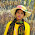declare
i number;
n number;
begin
i:=2;
n:=&a;
for i in 2..n/2 loop
if mod(n,i) <> 0 then
dbms_output.put_line(n||' is a prime number');
else
dbms_output.put_line(n||' is not a prime number');
end if;
end loop;
end;
/

2.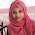declare
nm number(3);
begin
nm := &p;
for ij in 2..nm/2 loop
if mod(nm,ij)<>0 then
dbms_output.put_line(nm || 'is a prime number');
else
dbms_output.put_line(nm || 'is not a prime number');
end if;
end loop;
end;

3.declare
n number;
i number;
counter number;
begin
n:=&n;
i:=1;
counter:=0;
for i in 1..n loop
if mod(n,i)=0
then counter:=counter+1;
end if;
end loop;
if counter=2
then dbms_output.put_line(n||' is a prime No.');
else
dbms_output.put_line(n||' is a not prime No.');
end if;
end;

4.Id:201330499

declare
n number(10);
j number(10);
temp number(10):=1;
begin
n:=&number_for_prime_check;
for j in 2..n-1 loop
if(n mod j)=0 then
temp:=0;
exit;
end if;
end loop;
if(temp=1)then
dbms_output.put_line('Prime number');
else
dbms_output.put_line('Not prime number');
end if;
end;
/

5.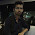ID : 201620195
https://www.dropbox.com/s/ui5m6pmfrs0np98/02.sql?dl=0

6.Md Aminul Islam Tushar
ID#201710063

7.Name: Yeasmin Sultana
ID#201710251

8.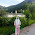Name:Sumsunnahar Nawa
ID:201710090

9.Md Rakibul Hasan
201720620
https://www.dropbox.com/s/042ayo12fko09dl/hw2q2.pdf?dl=0

10.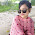ID: 201710404

11.id : 201710081
name : Eusha Chowdhury

12.abdul muzid sunny
id 201720450

13.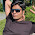count prime number from previously stored table value